## Plot conics and inequalities

The Functions application is now called the Grapher application and offers the ability to add expressions based on predefined templates. It is possible to add functions, equations of lines and conics, polar and parametric curves as well as inequalities.

To start graphing, all you have to do is select Add an element then press OK and choose a template. The Empty template allows you to type a freeform expression and the other templates can be modified.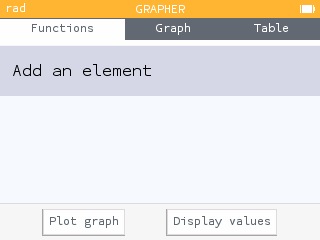It is thus possible to draw conics, surfaces defined by inequalities, polar and parametric curves, and equations of lines.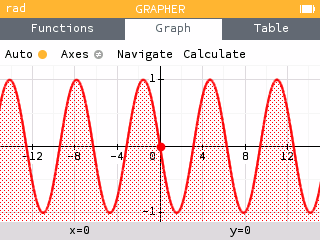For equations of lines, the menu with the three dots to the right of the expression allows you to obtain the slope and the y-intercept.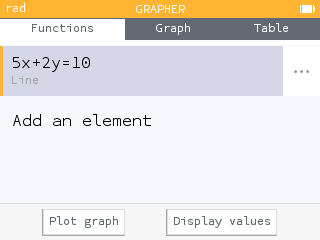For conics, the menu with the three dots to the right of the expression makes it possible to obtain the coordinates of the center, the semi-major axis, the semi-minor axis, and more.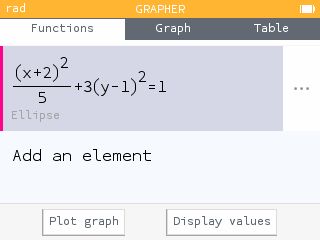## Statistical tests and confidence intervals

The Probability application is now called the Inference application and adds the ability to perform statistical tests and calculate confidence intervals.

When you enter the app, you can choose whether you want to calculate probabilities, perform hypothesis tests, or calculate confidence intervals.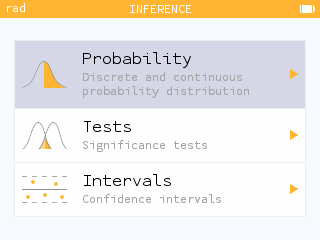You can perform a hypothesis tests on proportions or means. It is also possible to perform chi-square tests. Within a hypothesis test, input your parameters and statistics and quickly determine the conclusion of the test.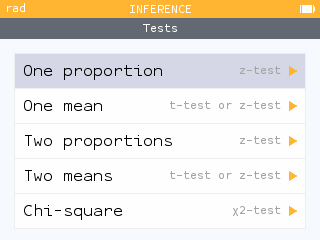The Intervals section allows you to calculate confidence intervals on a proportion, a mean, a difference of two proportions and a difference of two means.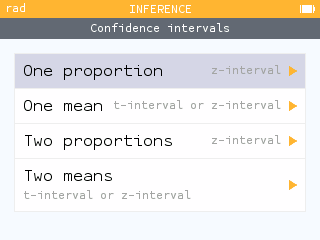## But wait, there's more!

This version also offers many other improvements.

Physics and Chemistry constants are now available in the toolbox.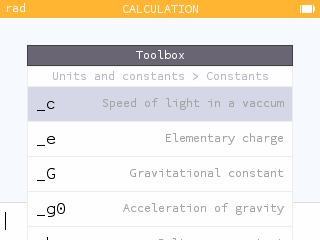The toolbox now opens with the last function used.

When calculating an integral in the Grapher application, the window dynamically adapts to the interval.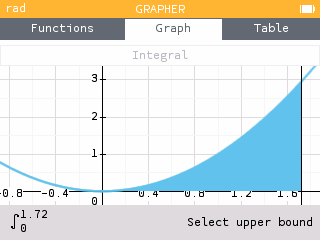It is now possible to reset the calculator from the Settings application.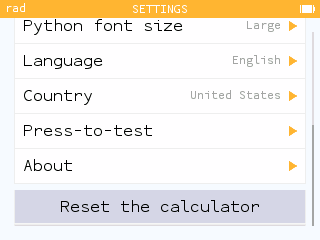Press-to-test allows you to deactivate features for class and exams.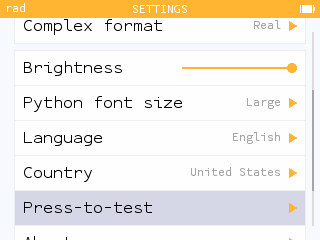• To clear the history of the Calculator application, you can press Shift then Backspace when the cursor is in the edit field.
• Radians are now the default angle unit.
• Units are now supported in calculations involving functions : `abs()`, `floor()`, `ceil()`, `round()` and `sign()`
• The alignment of superscripts and subscripts has been changed.
• A new examination mode for the International Baccalaureate (accessible when the country chosen is "International") is now available.